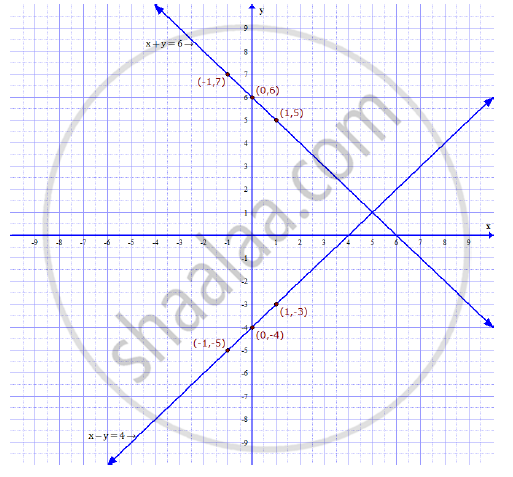SSC (Marathi Semi-English) 10thMaharashtra State Board
Share

# Solve the following simultaneous equations by using graphical method : x + y = 6;  x – y = 4. - SSC (Marathi Semi-English) 10th - Algebra

ConceptGraphical Method of Solution of a Pair of Linear Equations

#### Question

Solve the following simultaneous equations by using graphical method : x + y = 6;  x – y = 4.

#### Solution

First we find three solutions for each equation and make tables.
x + y = 6 ⇒ y = 6 – x
x – y = 4 ⇒ y = x – 4

 x+y=6 x 1 -1 0 y 5 7 6

Now, plot the points (1, 5), (–1, 7) and (0, 6) and draw the line passing through them

 x-y=4 x 1 -1 0 y -3 -5 -4

Now, plot the points (1, –3), (–1, –5) and (0, –4) and draw the line passing through them.Thus the point of intersection of two lines is (5, 1).
Hence x = 5 and y = 1 is the solution of the given equations.

Is there an error in this question or solution?

#### APPEARS IN

2014-2015 (March) (with solutions)
Question 3.3 | 3.00 marks
Solution Solve the following simultaneous equations by using graphical method : x + y = 6;  x – y = 4. Concept: Graphical Method of Solution of a Pair of Linear Equations.
S